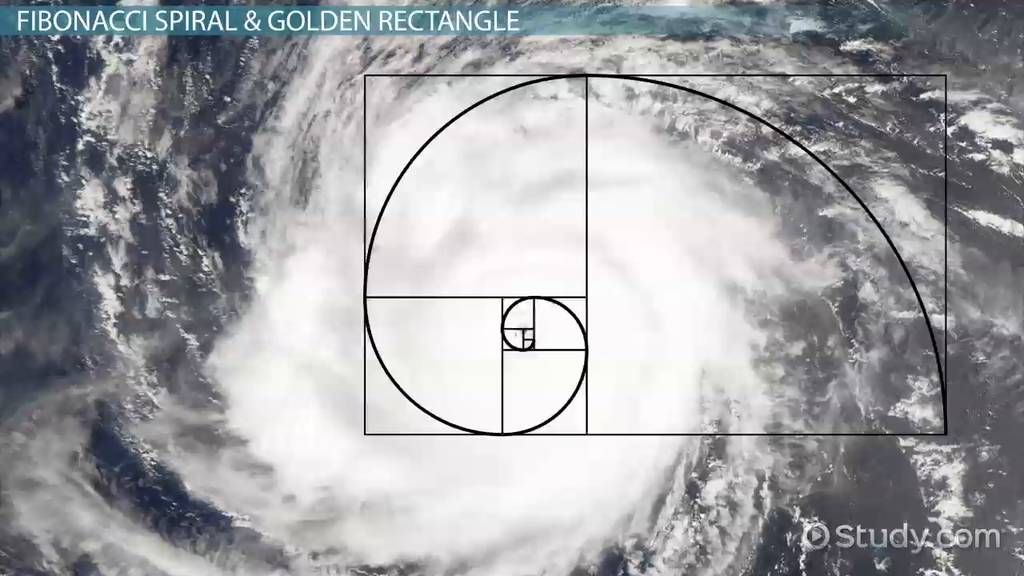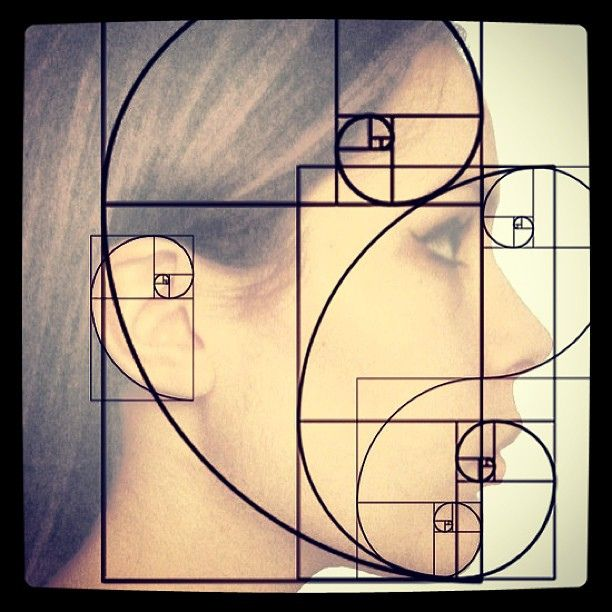# Nature By Numbers: Amazing Animated Fibonacci Sequence Will Leave You Speechless

0
4128

#### The Fibonacci sequence is a series of numbers where a number is found by adding up the two numbers before it. Starting with 0 and 1, the sequence goes 0, 1, 1, 2, 3, 5, 8, 13, 21, 34, and so forth. Written as a rule, the expression is xn = xn-1 + xn-2.

Fibonacci numbers do actually appear in nature, from sunflowers to hurricanes to galaxies. Sunflowers seeds, for example, are arranged in a Fibonacci spiral, keeping the seeds uniformly distributed no matter how large the seed head may be.A Fibonacci spiral is a series of connected quarter-circles drawn inside an array of squares with Fibonacci numbers for dimensions. The squares fit perfectly together because of the nature of the sequence, where the next number is equal to the sum of the two before it.

Any two successive Fibonacci numbers have a ratio very close to the Golden Ratio, which is roughly 1.618034. The larger the pair of Fibonacci numbers, the closer the approximation. The spiral and resulting rectangle are known as the Golden Rectangle.

You can take a walk in a park and find this pattern on plants and bushes quite easily.

Many flowers offer a beautiful confirmation of the Fibonacci mystique. A daisy has a central core consisting of tiny florets arranged in opposing spirals. There are usually 21 going to the left and 34 to the right.

A mountain aster may have 13 spirals to the left and 21 to the right. Sunflowers are the most spectacular example, typically having 55 spirals one way and 89 in the other; or, in the finest varieties, 89 and 144.

Pine cones are also constructed in a spiral fashion, small ones having commonly with 8 spirals one way and 13 the other. The most interesting is the pineapple – built from adjacent hexagons, three kinds of spirals appear in three dimensions. There are 8 to the right, 13 to the left, and 21 vertically – a Fibonacci triple.

Why should this be? Why has Mother Nature found an evolutionary advantage in arranging plant structures in spiral shapes exhibiting the Fibonacci sequence?